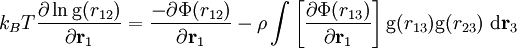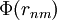Born-Green equation$k_B T \frac{\partial \ln {\rm g}(r_{12})}{\partial {\mathbf r}_1}= \frac{-\partial \Phi(r_{12})}{\partial {\mathbf r}_1}- \rho \int \left[ \frac{\partial \Phi(r_{13})}{\partial {\mathbf r}_1} \right] {\rm g}(r_{13}){\rm g}(r_{23}) ~ {\rm d}{\mathbf r}_3$
where$\Phi(r_{nm})$ is the intermolecular pair potential, T is the temperature, and$k_B$ is the Boltzmann constant.